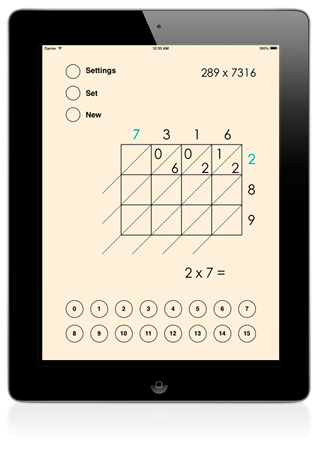# Lattice Multiplication

### for iPad, iPhone, Mac, and Windows 10 PCs

This app can be used to teach and study the ancient lattice multiplication method. Solving lattice multiplication problems is also excellent times tables practice. The app is very easy to use and it has an intuitive interface with customizable colors and other settings. The user can solve random and custom multiplication problems with small and large numbers.

##The lattice multiplication method

Lattice multiplication is algorithmically equivalent to long multiplication. A lattice (a grid) guides the calculation. All the multiplications are done first and then the additions. The method was introduced to Europe in 1202 in Fibonacci's Liber Abaci.

In this groundbreaking book Fibonacci presented many algorithms for working with Arabic numerals. Ancient Indians and Chinese originally invented some of the algorithms. Fibonacci presented both the current standard long multiplication and also an originally Indian method called lattice multiplication, which is faster and more compact for working with larger numbers.

## The Lattice Multiplication app

The Lattice Multiplication app allows the user to solve a lattice multiplication problem step by step and animates all the steps. In the steps the user will multiply or add. The correct answer will fly to the right place. If the user presses the wrong button the answer will appear above the keyboard but it will not move.

## Settings

• The multiplicand can have up to 5 digits
• The multiplier can have up to 3 digits
• The current operation for each step can be hidden
• The operands for the current operation can be highlighted
• There are 3 different themes: black, gray and gold
• The speed of the animations can be set.

54 x 73

567 x 66439

## Lattice Multiplication in the Apple VPP Store for Education

The Volume Purchase Program allows participating educational institutions to purchase iDevBooks math apps in volume and distribute them to students. All iDevBooks math apps offer special 50% discount for purchases of 20 apps or more for participating educational institutions.

Lattice Multiplicaton in the VPP store